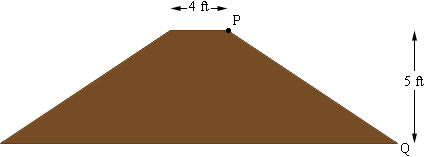SEARCH HOMEMath Central Quandaries & QueriesQuestion from Katherine: I need to know how to figure the acreage a berm might cover. Berm size is 815 ft. long with a 5' height average at a 3:1 slope It will have a 4 ft. top. Also how do you figure how wide a base would be for a berm at a 3:1 slope? I am thinking for every 3 ft horizontal I need to go 1 foot vertical starting from the center out. Is this only half the berm and need to mirror it for the other side to get a total base width?Hi Katherine,

Here is a cross section of the berm you describe (not to scale).Suppose you are at the point P and you walk down the slope towards Q. For every 1 foot you go vertically you go 3 feet horizontally. You need to go 5 feet vertically to arrive at Q and hence you have gone $3 \times 5 = 15$ feet horizontally. The same is true for the slope on the other side of the berm so its width at the base is $15 + 4 + 15 = 34$ feet. Hence the berm covers a rectangle of ground that is 815 feet by 34 feet and thus the area is $815 \times 34 = 27710$ square feet. There are 43560 square feet in an acre so the berm covers $\large \frac{27710}{43560} = 0.64$ acres.

PennyMath Central is supported by the University of Regina and The Pacific Institute for the Mathematical Sciences.